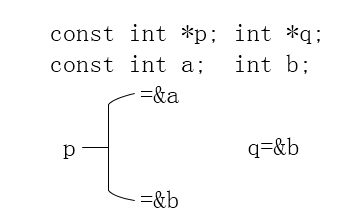代码回顾：从一个文本文件中读取一行的内容，进行数据处理。

   <pre name="code" class="html"> string tmpStr("");
string *a = new string[MAX_LINES];
int index = 0;
inFile.open("haha.txt", ios::in);
if (NULL == inFile)
{
cout << "文件打开失败！" << endl;
return ;
}
while (<span style="font-family: Arial, Helvetica, sans-serif;">getline(inFile, tmpStr)</span>)
{
a[index] = tmpStr;
index += 1;
}
for (int j = 0; j < index; j++)
{
cout << a[j] << endl;
}
//string 转换为char
const char *p = a->c_str();
char *pData;
pData=const_cast<char *>(p);
preDealTheUartData_Purifier(( uint8_t *)p,strlen(p));

1、

static void  preDealTheUartData_Purifier(  uint8_t *data, uint32_t len);

void funA(int *a);
void funB(const int *b);
int *ia;
const int *ib;
funB(ia);    //正确
funB(ib);    //正确
funA(ia);    //正确
funA(ib);    //错误：不可以将非const对象的指针指向const对象，但是可以将const对象的指针指向非const对象2、

getline(inFile, tmpStr)

size_t getline(char **lineptr, size_t *n, FILE *stream);

<span style="white-space:pre">	</span>FILE *fp;
char *ch=new char[MAX_SIZE];
if((fp=fopen("haha.txt","ab+"))==NULL)    //ab+以二进制打开,可读写
{
printf("\n不能打开文件!");
return 0;
}
ch[len]=fgetc(fp);     //存入数组
while(ch[len]!=EOF)    //读到文件末尾
{
if(len<MAX_SIZE)
ch[++len]=fgetc(fp);
else
{
printf("\n输入的数据超过界限!");
return 0;
}
}